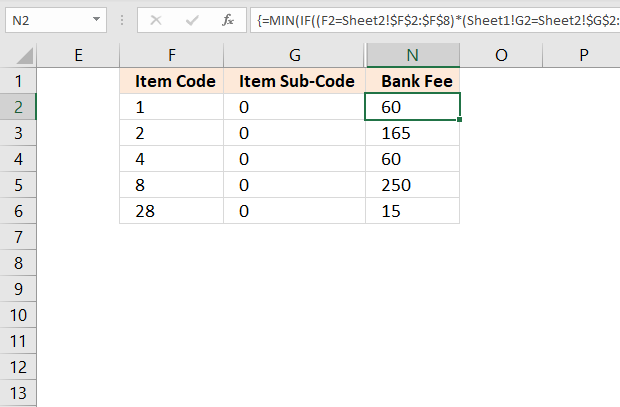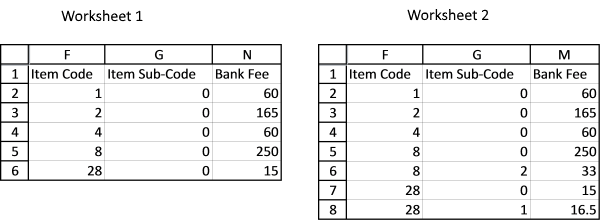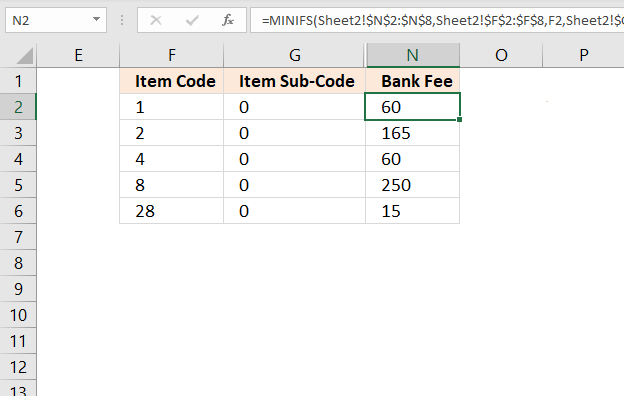Author: Oscar Cronquist Article last updated on February 09, 2023This article describes an array formula that compares values from two different columns in two worksheets twice and returns a value if criteria are met.

Hi Oscar,
There are multiple columns in two different worksheets, one has more columns than another. I need to compare column F of worksheet 1 and column E of worksheet 2; if the value matches, compare column G of worksheet 1 and column F of worksheet 2; if the value matches, record the value in column N of worksheet1 from column M of worksheet 2. Please see the example below.
Worksheet 1:
Column F Column G Column N
Item Code Item Sub-Code Bank Fee
1 0
2 0
4 0
8 0
28 0
Worksheet2:
Column E Column F Column M
Item Code Item Sub-Code Bank Fee
1 0 60
2 0 165
4 0 60
8 0 250
8 2 33
28 0 15
28 1 16.5Array formula in cell N2, worksheet 1:

=MIN(IF((F2=Sheet2!\$F\$2:\$F\$8)*(Sheet1!G2=Sheet2!\$G\$2:\$G\$8), Sheet2!\$N\$2:\$N\$8, ""))

## 1.1 How to enter an array formula

1. Copy and paste the formula above to cell N2.
2. Press and hold CTRL + SHIFT simultaneously.
3. Press Enter once.
4. Release all keys.

The formula is now surrounded by curly brackets, like this {=formula} if you did it right. Check your formula bar and make sure you have the curly brackets.

Then copy cell N2 and paste to cells below.

## 1.2 Explaining array formula in cell N2

#### Step 1 - Compare cell F2 (sheet1) with column F (sheet2)

The equal sign is a logical operator that allows you to compare values. It also allows you to compare a value to multiple values, this returns an array of values.

F2=Sheet2!\$F\$2:\$F\$8

becomes

1={1;2;4;8;8;28;28}

and returns

{TRUE; FALSE; FALSE; FALSE; FALSE; FALSE; FALSE}

TRUE and FALSE are boolean values.

#### Step 2 - Compare cell G2 (sheet1) with column G (sheet2)

G2=Sheet2!\$G\$2:\$G\$8

becomes

0={0;0;0;0;2;0;1}

and returns

{TRUE; TRUE; TRUE; TRUE; FALSE; TRUE; FALSE}

#### Step 3 - Multiply arrays

This step multiples both arrays to apply AND-logic meaning both boolean values must be TRUE in order to return TRUE. See all possible combinations below.

TRUE * TRUE = TRUE (1)

TRUE * FALSE = FALSE (0)

FALSE * TRUE = FALSE (0)

FALSE * FALSE = FALSE (0)

(F2=Sheet2!\$F\$2:\$F\$8)*(Sheet1!G2=Sheet2!\$G\$2:\$G\$8)

becomes

({TRUE; FALSE; FALSE; FALSE; FALSE; FALSE; FALSE}) * ({TRUE; TRUE; TRUE; TRUE; FALSE; TRUE; FALSE})

and returns {1; 0; 0; 0; 0; 0; 0}.

The calculation returns the numerical equivalent to the boolean values, TRUE returns 1, and FALSE returns 0 (zero).

#### Step 4 - Use IF function to replace calculated values with sheet2 values from column N, if TRUE (1)

The IF function returns one value if the logical test is TRUE and another value if the logical test is FALSE.

IF(logical_test, [value_if_true], [value_if_false])

IF((F2=Sheet2!\$F\$2:\$F\$8)*(Sheet1!G2=Sheet2!\$G\$2:\$G\$8), Sheet2!\$N\$2:\$N\$8, "")

becomes

IF({1;0;0;0;0;0;0}, Sheet2!\$N\$2:\$N\$8, "")

becomes

IF({1; 0; 0; 0; 0; 0; 0}, {60; 165; 60; 250; 33; 15; 16.5}, "")

and returns {60; ""; ""; ""; ""; ""; ""}.

#### Step 5 - Calculate the smallest number in the array

The MIN function returns the smallest number in an array or cell range, it ignores logical and text values

MIN(IF((F2=Sheet2!\$F\$2:\$F\$8)*(Sheet1!G2=Sheet2!\$G\$2:\$G\$8), Sheet2!\$N\$2:\$N\$8, ""))

becomes

MIN({60;"";"";"";"";"";""})

and returns 60.

## 2. Compare two columns in different worksheets (Excel 2016)Excel 2016 formula in cell N2:

=MINIFS(Sheet2!\$N\$2:\$N\$8, Sheet2!\$F\$2:\$F\$8, F2, Sheet2!\$G\$2:\$G\$8, G2)

### Explaining formula in cell N2

#### Step 1 - Setup MINIFS function

The MINIFS function calculates the smallest value based on a given set of criteria.

MINIFS(min_rangecriteria_range1criteria1, [criteria_range2criteria2], ...)

MINIFS(Sheet2!\$N\$2:\$N\$8, Sheet2!\$F\$2:\$F\$8, F2, Sheet2!\$G\$2:\$G\$8, G2)

#### Step 2 - Evaluate MINIFS function

MINIFS(Sheet2!\$N\$2:\$N\$8, Sheet2!\$F\$2:\$F\$8, F2, Sheet2!\$G\$2:\$G\$8, G2)

becomes

MINIFS({60;165;60;250;33;15;16.5},{1;2;4;8;8;28;28},1,{0;0;0;0;2;0;1},0)

and returns 60. The only relative position that meets both criteria is the first one, it contains number 60.